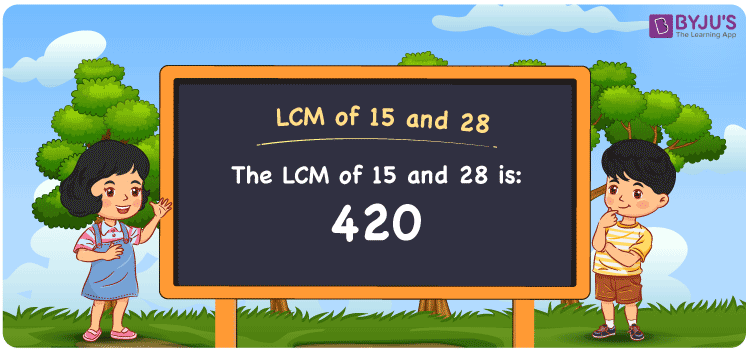# LCM of 15 and 28

LCM of 15 and 28 is 420.The Least Common Multiple or Lowest Common Multiple known as LCM is the smallest number that is divisible by the given numbers. In the given set of numbers 15 and 28, 420 is the first(least or smallest) number that is common in the set of multiples of 15 and 28. The LCM is also known as LCD, Least Common Divisor. You can refer to LCM for all details.

## What is LCM of 15 and 28

The Least Common Multiple or Lowest Common Multiple of 15 and 28 is 420.## How to Find LCM of 15 and 28?

LCM of 15 and 28 can be determined using three methods:

• Prime Factorisation
• Division method
• Listing the multiples

### LCM of 15 and 28 Using Prime Factorisation Method

In the Prime Factorisation method, the numbers 15 and 28 can be expressed as;

15 = 3 × 5

28 = 2 × 2 × 7

Since there are no common prime factors, the product of all the prime factors is the LCM.

LCM (15, 28) = 2 × 2 × 3 × 5 × 7 = 420

### LCM of 15 and 28 Using Division Method

In the Division Method, the given numbers 15 and 28 are divided together. Since there are no common prime divisors, the product of all the common prime factors of 15 and 28 is the LCM.

 2 15 28 2 15 14 3 15 7 5 5 7 7 1 7 × 1 1

Hence, LCM (15, 28) = 2 × 2 × 3 × 5 × 7 = 420

### LCM of 15 and 28 Using Listing the Multiples

By listing all the multiples of 15 and 28, we can identify the LCM. Below is the list of multiples for 15 and 28

Multiples of 15

15, 30, 45, 60, 75, 90, 105, 120, 135, 150, 165, 180, 195, 210, 225, 240, 255, 270, 285, 300, 315, 330, 345, 360, 375, 390, 405, 420, 435, 450

Multiples of 28

28, 56, 84, 112, 140, 168, 196, 224, 252, 280, 308, 336, 364, 392, 420, 448, 476

LCM (15, 28) = 420

## Video Lesson on Applications of LCM## Solved Examples

What is the smallest number that is divisible by both 15 and 28?

Answer: 420 is the smallest number that is divisible by both 15 and 28.

What is the LCM for 5, 15 and 28?

Answer: LCM for 5, 15 and 28 is 420 as 5 is the prime factor of 15.

## Frequently Asked Questions on LCM of 15 and 28

### What is the LCM of 15 and 28?

The LCM of 15 and 28 is 420.

### Is the LCM of 15 and 28 the same as the HCF of 15 and 28?

No. Least Common Multiple of 15 and 28 is 420 and Highest Common Factors of 15 and 28 is 1.

### What is the common factor between 15 and 28?

1 is the common factor in the numbers 15 and 28.

### What are the first 3 common multiples of 15 and 28?

The first three common multiples of 15 and 28 are 420, 840, and 1260.

### Is the LCM of 5 and 15 same as the LCM of 7 and 28?

No. LCM of 5 and 15 is 15, whereas the LCM of 7 and 28 is 28. They are not the same.